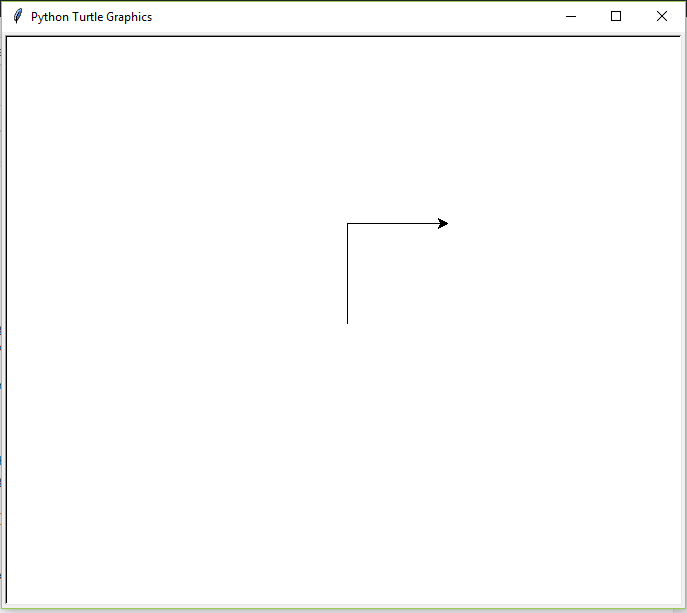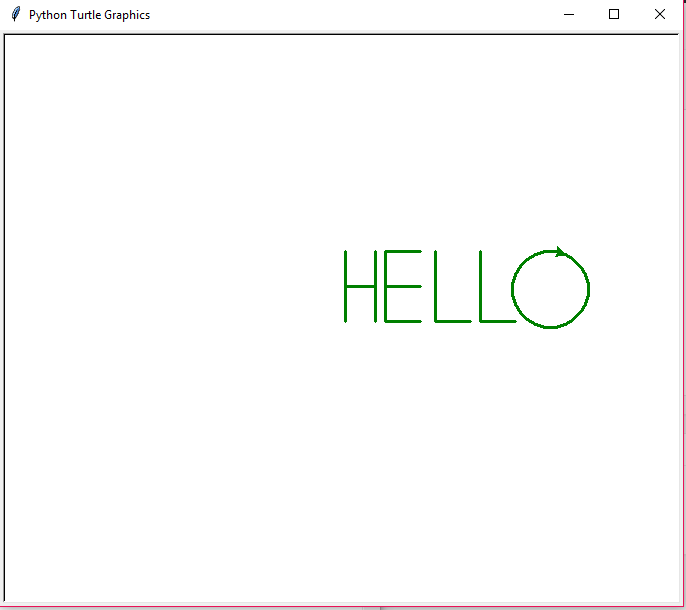# Printing HELLO with turtle module

Turtle is a Python Module which allows us to draw a various geometrical illustration by just importing it in Python and using the inbuilt function of the turtle Module. We can use functions like turtle.forward(…) and turtle.right(…) which can move the turtle around. Turtle is a beginner-friendly way to learn python by running some basic commands and viewing the turtle do it graphically. It is like a drawing board that allows you to draw over it. The turtle module can be used in both object-oriented and procedure-oriented ways.

Turtle methods used are as follows:

#### Function used for knowing the turtle’s state:

position()– This function is used to get the coordinates/position of the turtle pointer. No parameter is required here as this function is not used for giving input but is used to output the position of turtle in the console window.

Example:

## python3

 `# Python program to` `# demonstrate basics of turtle`   `# Importing and making a turtle object` `# with background color as white` `import` `turtle`   `frame ``=` `turtle.Screen().bgcolor(``"White"``)` `draw ``=` `turtle.Turtle()` `draw.left(``90``)` `draw.forward(``100``)` `draw.right(``90``)` `draw.forward(``100``)`

Output:After having learned the basics of turtle, let’s write the code for printing ‘HELLO’. Below is the implementation.

## python3

 `# Python program to` `# demonstrate printing HELLO` `# using turtle`   `# Here frame is initialized with` `# background colour as "White"` `import` `turtle` `frame ``=` `turtle.Screen().bgcolor(``"White"``)` `draw ``=` `turtle.Turtle()`   `# The colour, width and speed of the pen is initialized` `draw.color(``"Green"``)` `draw.width(``3``)` `draw.speed(``10``)`   `# Now lets get started with actual code` `# printing letter H` `draw.left(``90``)` `draw.forward(``70``)` `draw.penup()` `draw.goto(``0``, ``35``)` `draw.pendown()` `draw.right(``90``)` `draw.forward(``30``)` `draw.penup()` `draw.goto(``30``, ``70``)` `draw.pendown()` `draw.right(``90``)` `draw.forward(``70``)`   `# printing letter E` `draw.penup()` `draw.goto(``40``, ``0``)` `draw.pendown()` `draw.right(``180``)` `draw.forward(``70``)` `draw.right(``90``)` `draw.forward(``35``)` `draw.penup()` `draw.goto(``40``, ``35``)` `draw.pendown()` `draw.forward(``35``)` `draw.penup()` `draw.goto(``40``, ``0``)` `draw.pendown()` `draw.forward(``35``)`   `# printing letter L` `draw.penup()` `draw.goto(``90``, ``70``)` `draw.pendown()` `draw.right(``90``)` `draw.forward(``70``)` `draw.left(``90``)` `draw.forward(``35``)`   `# printing letter L` `draw.penup()` `draw.goto(``135``, ``70``)` `draw.pendown()` `draw.right(``90``)` `draw.forward(``70``)` `draw.left(``90``)` `draw.forward(``35``)`   `# printing letter O` `draw.penup()` `draw.goto(``210``, ``70``)` `draw.pendown()` `for` `i ``in` `range``(``25``):` `    ``draw.right(``15``)` `    ``draw.forward(``10``)`

Output:Whether you're preparing for your first job interview or aiming to upskill in this ever-evolving tech landscape, GeeksforGeeks Courses are your key to success. We provide top-quality content at affordable prices, all geared towards accelerating your growth in a time-bound manner. Join the millions we've already empowered, and we're here to do the same for you. Don't miss out - check it out now!

Previous
Next